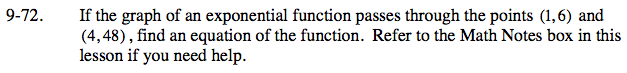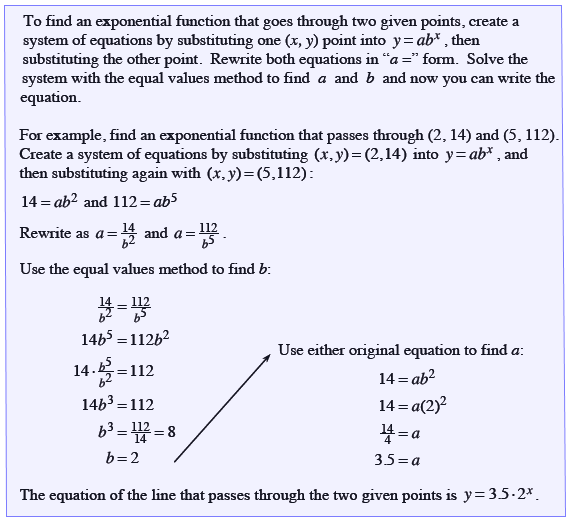### Home > CCA > Chapter 9 > Lesson 9.3.1 > Problem9-72

9-72.

If the graph of an exponential function passes through the points (1, 6) and (4, 48), find an equation of the function. Refer to the Math Notes box in this lesson if you need help. Homework Help ✎Use the Math Notes box for help.

a = 3